RD Sharma Class 12 Solutions Chapter 13 Derivative as a Rate Measurer Ex 13.2 are part of RD Sharma Class 12 Solutions. Here we have given RD Sharma Class 12 Solutions Chapter 13 Derivative as a Rate Measurer Ex 13.2.

Here you can get free RD Sharma Solutions for Class 12 Maths of Chapter 13 Derivative as a Rate Measurer Exercise 13.2. All RD Sharma Book Solutions are given here exercise wise for Derivative as a Rate Measurer. RD Sharma Solutions are helpful in the preparation of several school level, graduate and undergraduate level competitive exams. Practicing questions from RD Sharma Mathematics Solutions for Class 12 Chapter 13 Derivative as a Rate Measurer is proven to enhance your math skills.

 Class: 12th Class Chapter: Chapter 13 Name: Derivative as a Rate Measurer Exercise: Exercise 13.2

## RD Sharma Class 12 Solutions Chapter 13 Derivative as a Rate Measurer Ex 13.2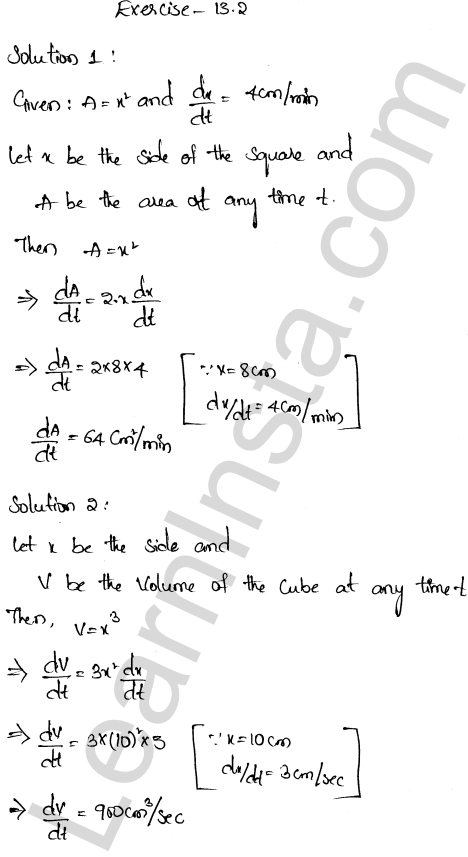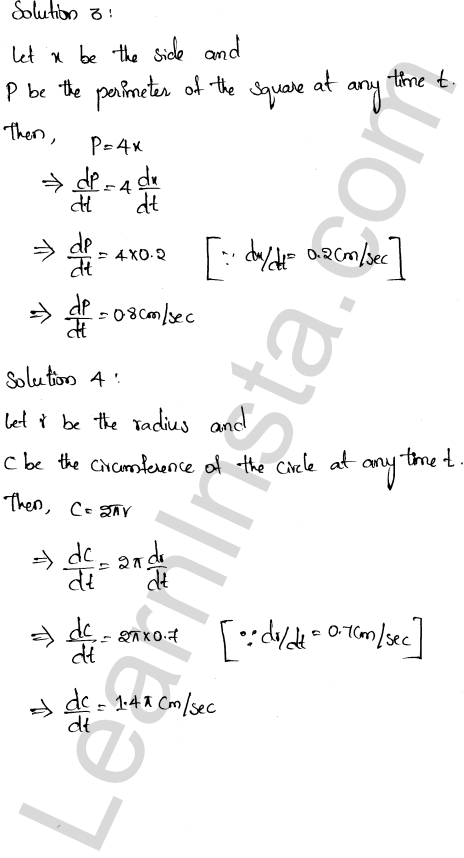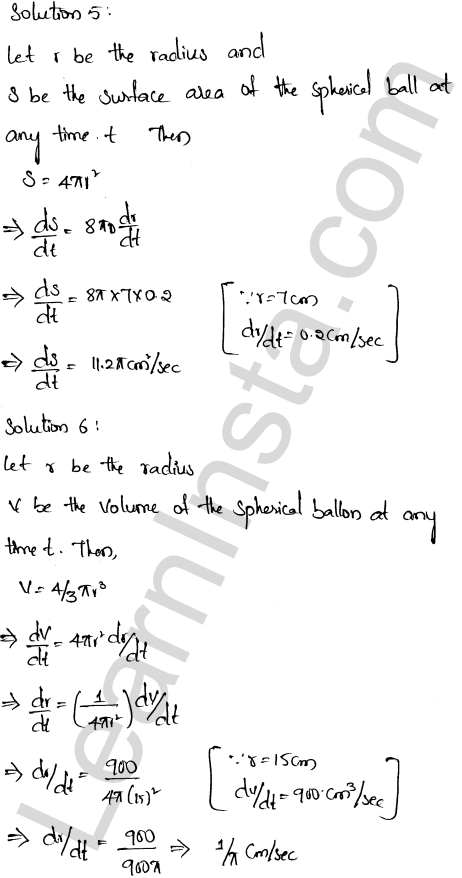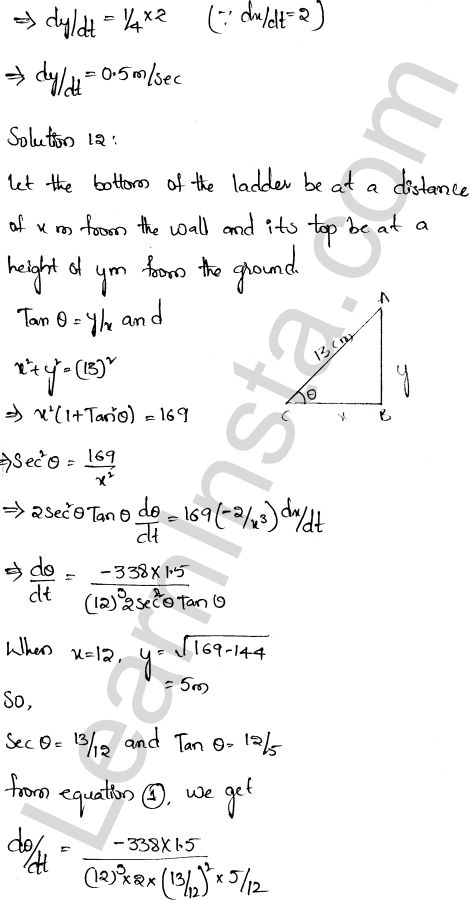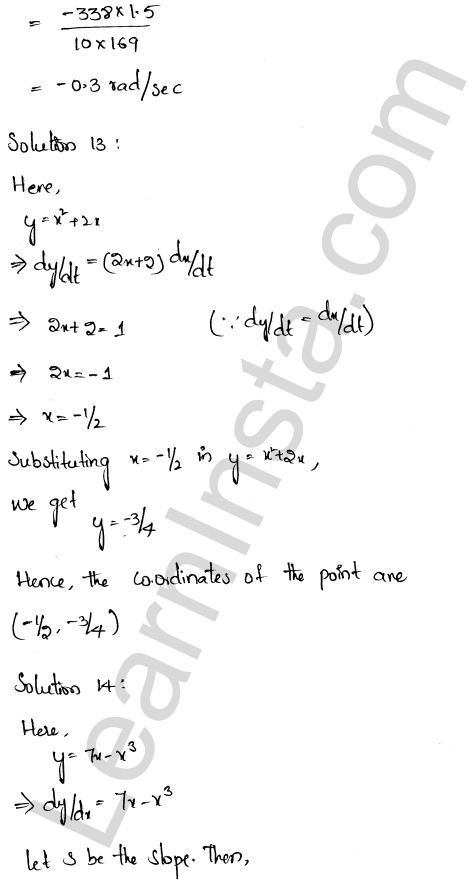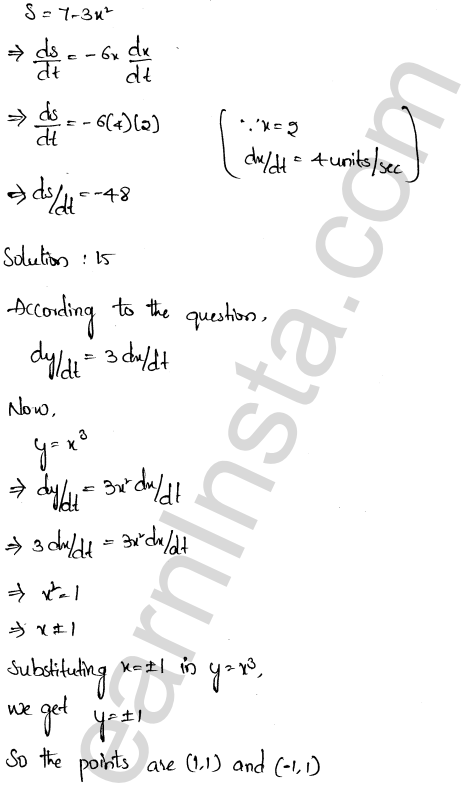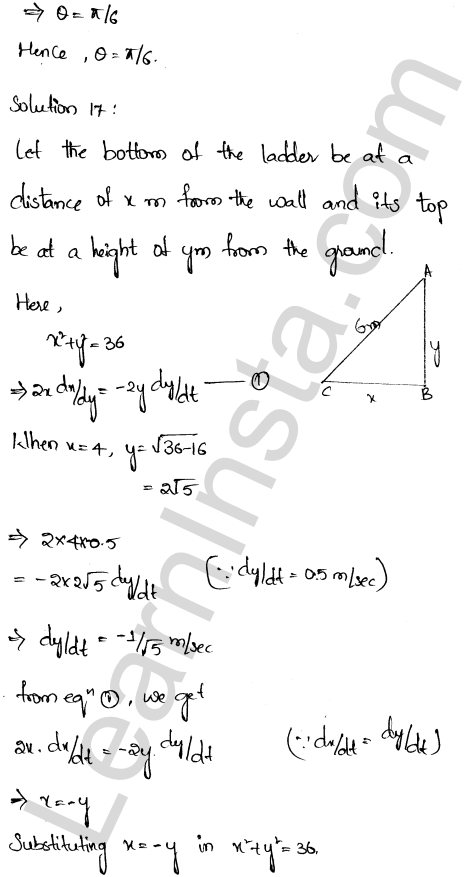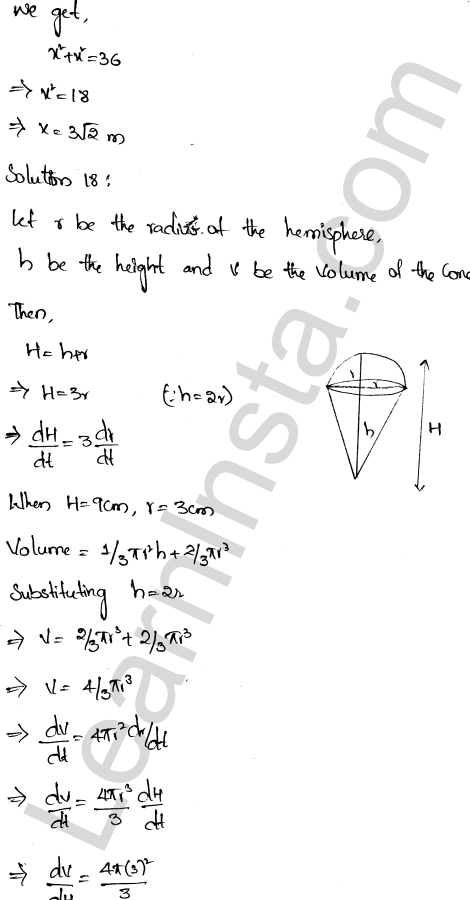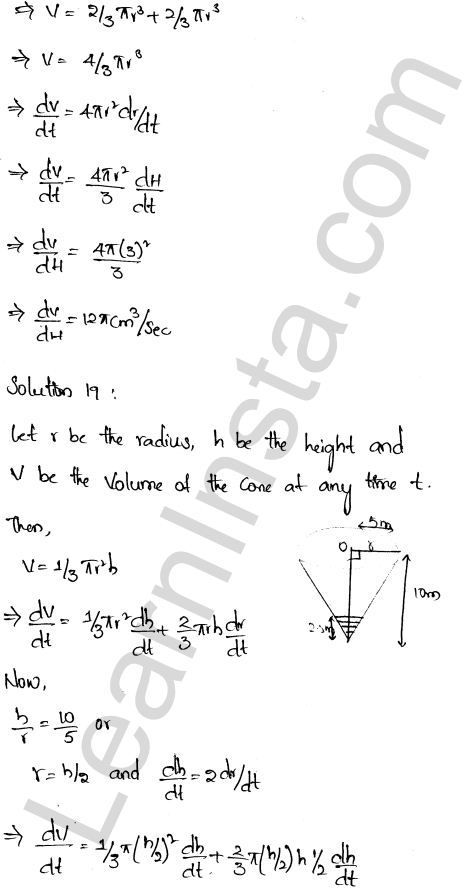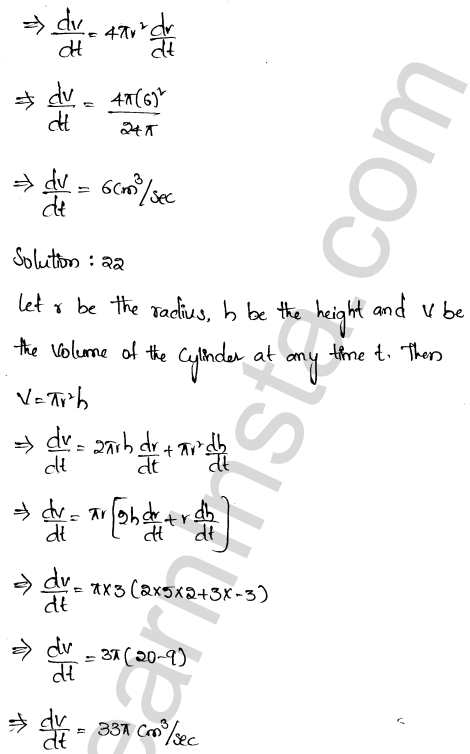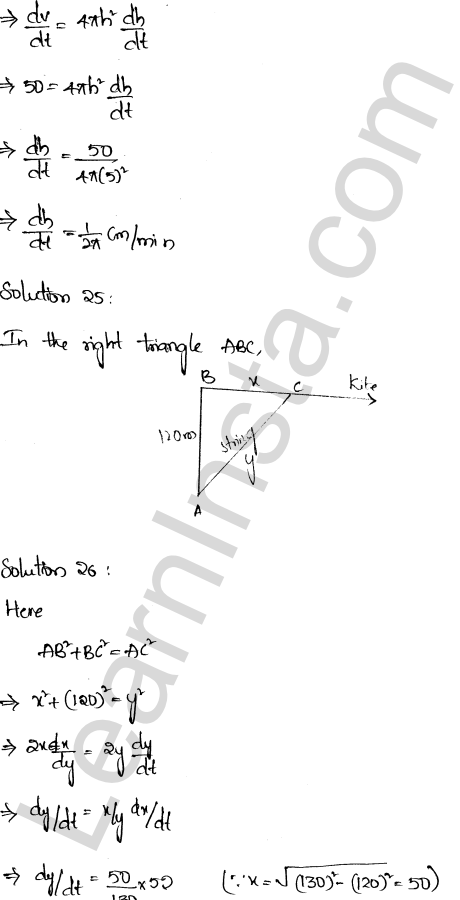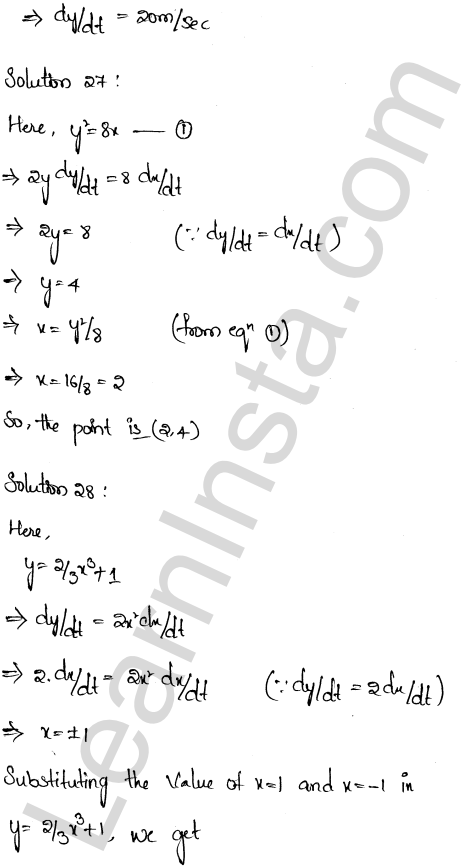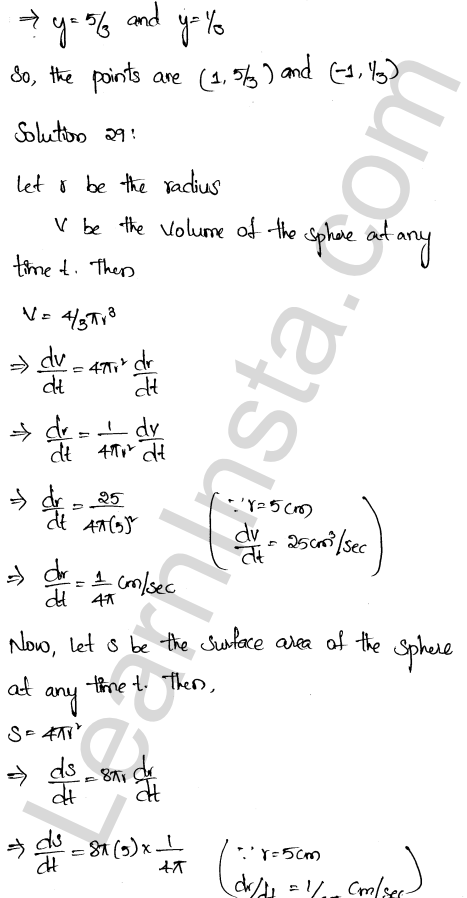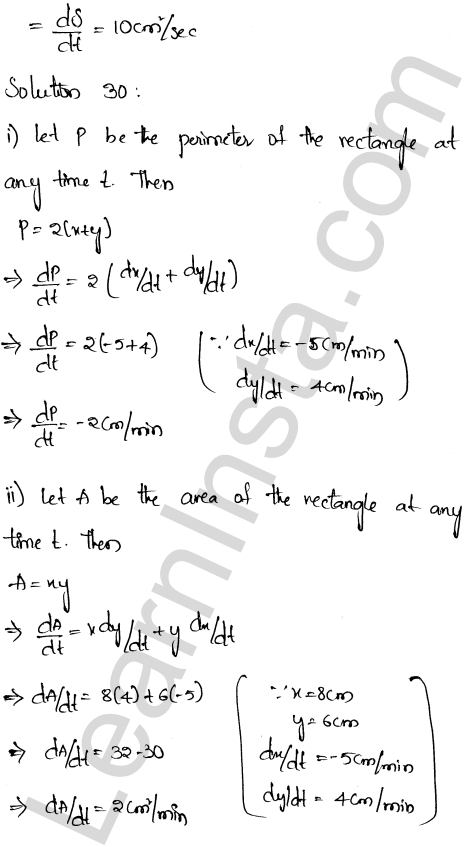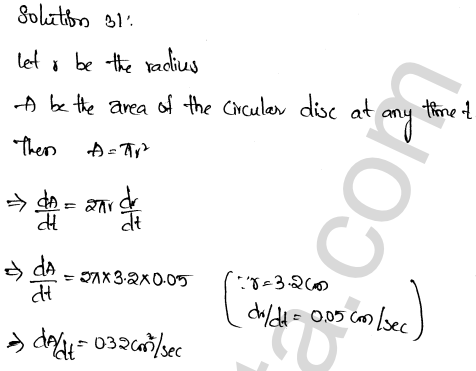We hope the RD Sharma Class 12 Solutions Chapter 13 Derivative as a Rate Measurer Ex 13.1, help you. If you have any query regarding RD Sharma Class 12 Solutions Chapter 13 Derivative as a Rate Measurer Ex 13.1, drop a comment below and we will get back to you at the earliest.